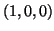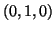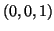Next: Root variable Up: Dimension, variable and attribute Previous: MINC general attributes   Contents

## Dimensions and dimension variables

The following are the names of dimensions and their corresponding dimension variables. Only MIvector_dimension does not have a corresponding dimension variable.

MIxspace
Dimension and coordinate variable for x axis.
MIyspace
Dimension and coordinate variable for y axis.
MIzspace
Dimension and coordinate variable for z axis.
MItime
Dimension and coordinate for variable time axis.
MItfrequency
Dimension and coordinate variable for temporal frequency.
MIxfrequency
Dimension and coordinate variable for spatial frequency along the x axis.
MIyfrequency
Dimension and coordinate variable for spatial frequency along the y axis.
MIzfrequency
Dimension and coordinate variable for spatial frequency along the z axis.
MIvector_dimension
Dimension only for components of a vector field.

In addition to dimension variables, we can have dimension width variables that specify the FWHM width of the sample at each point.

MIxspace_width
Width of samples along x axis.
MIyspace_width
Width of samples along y axis.
MIzspace_width
Width of samples along z axis.
MItime_width
Width of samples along time axis.
MItfrequency_width
Width of samples along temporal frequency axis.
MIxfrequency_width
Width of samples along x spatial frequency axis.
MIyfrequency_width
Width of samples along y spatial frequency axis.
MIzfrequency_width
Width of samples along z spatial frequency axis.

The attributes associated with dimension and dimension width variables are given below:

MIspacing
A string with value MI_REGULAR (for a regularly spaced grid) or MI_IRREGULAR (for irregular spacing of samples). If the value is MI_REGULAR, then the variable is permitted to be a scalar instead of a vector varying with the dimension of the same name. Can be used for both dimension and dimension width variables.
MIstep
A number indicating the step between samples for regular spacing. This value may be negative to indicate orientation (to specify that MIxspace runs from patient right to left, for example). If the spacing is irregular, then the value can be an average step size. Applies only to dimension variables.
MIstart
The coordinate of the index 0 of the dimension. Applies only to dimension variables.
MIspacetype
A string identifying the type of coordinate space. Currently one of MI_NATIVE (the coordinate system of the scanner), MI_TALAIRACH (a standardized coordinate system), or MI_CALLOSAL (another standardized coordinate system). Applies only to dimension variables.
MIalignment
A string indicating the position of the coordinates relative to each sample (remembering that each sample has a width). Can be one of MI_START, MI_CENTRE or MI_END. Applies only to dimension variables.
MIdirection_cosines
A vector with MI_NUM_SPACE_DIMS(=3) elements giving the direction cosines of the axes. Although axes are labelled x, y and z, they may in fact have a signficantly different direction -- this attribute allows the direction relative to the true axes to be specified exactly. Applies only to dimension variables. Note that the dot product of the direction cosine with the corresponding axis (,or) should be positive so that the sign of the attribute MIstep contains the orientation information.
MIwidth
For regularly dimension widths, this numeric attribute gives the FWHM width of all samples. It can be used for irregular widths to specify the average width. Applies only to dimension width variables.
MIfiltertype
A string specifying the shape of the convolving filter. Currently, can be one of MI_SQUARE, MI_GAUSSIAN or MI_TRIANGULAR. Applies only to dimension width variables.Next: Root variable Up: Dimension, variable and attribute Previous: MINC general attributes   Contents
Robert VINCENT 2004-05-28